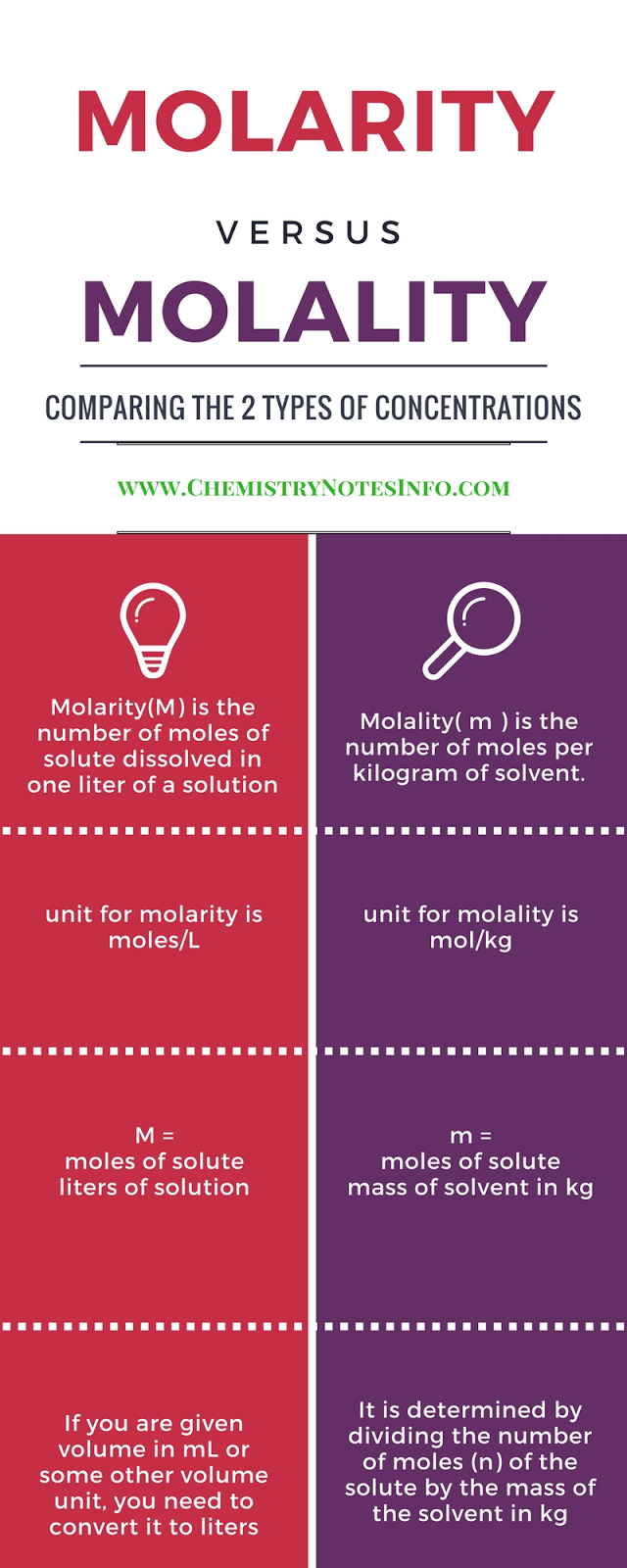## What is molarity?

Molarity is also known as molar concentration, it is the ratio of moles of substance to volume in litre.
Where mole is weight in gram devided by molecular weight.
Molarity is chemistry terminology.

### Molarity (M) :-

No. of moles of the solute  / Volume of the solution in litre.
M = strength in gram per litre / Molar mass of the solute
Unit =>   M = Moles/LWhat is Molarity